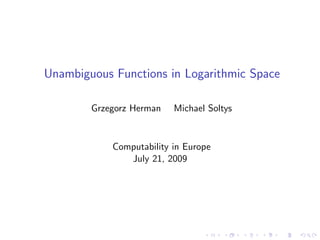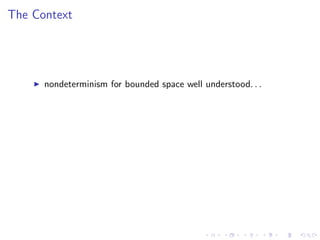Successfully reported this slideshow.

# Unambiguous functions in logarithmic space - CiE 2009×

1 of 34
1 of 34

# Unambiguous functions in logarithmic space - CiE 2009

## More Related Content

### Unambiguous functions in logarithmic space - CiE 2009

1. 1. Unambiguous Functions in Logarithmic Space Grzegorz Herman Michael Soltys Computability in Europe July 21, 2009
2. 2. The Context
3. 3. The Context nondeterminism for bounded space well understood. . .
4. 4. The Context nondeterminism for bounded space well understood. . . except L vs. NL (since 2004, SL vs. NL)
5. 5. The Context nondeterminism for bounded space well understood. . . except L vs. NL (since 2004, SL vs. NL) unambiguity seems to be the most useful intermediate step
6. 6. The Context nondeterminism for bounded space well understood. . . except L vs. NL (since 2004, SL vs. NL) unambiguity seems to be the most useful intermediate step a breakthrough due to Reinhardt and Allender (1997): UL/poly = NL/poly
7. 7. The Context nondeterminism for bounded space well understood. . . except L vs. NL (since 2004, SL vs. NL) unambiguity seems to be the most useful intermediate step a breakthrough due to Reinhardt and Allender (1997): UL/poly = NL/poly no major results since
8. 8. Rationale
9. 9. Rationale want to measure relative (un)ambiguity of problems
10. 10. Rationale want to measure relative (un)ambiguity of problems need a meaningful notion of unambiguous nondeterministic reductions
11. 11. Rationale want to measure relative (un)ambiguity of problems need a meaningful notion of unambiguous nondeterministic reductions need a well-behaved model for computing functions
12. 12. Nondeterministic Function Classes: Existing Models
13. 13. Nondeterministic Function Classes: Existing Models multi-valued functions, or
14. 14. Nondeterministic Function Classes: Existing Models multi-valued functions, or functions expressing properties of computation graphs (e.g., #L, GapL), or
15. 15. Nondeterministic Function Classes: Existing Models multi-valued functions, or functions expressing properties of computation graphs (e.g., #L, GapL), or deterministic computation with oracle queries (e.g., FNL = FLNL).
16. 16. Nondeterministic Function Classes: Our Model
17. 17. Nondeterministic Function Classes: Our Model nondeterministic machines with deterministic answers
18. 18. Nondeterministic Function Classes: Our Model nondeterministic machines with deterministic answers oracle-based input and output
19. 19. Nondeterministic Function Classes: Our Model nondeterministic machines with deterministic answers oracle-based input and output explicit failures (uncatchable exceptions)
20. 20. Nondeterministic Function Classes: Our Model nondeterministic machines with deterministic answers oracle-based input and output explicit failures (uncatchable exceptions) (un)ambiguity captured by the shape of computation graphs
21. 21. Reductions: The Deﬁnition
22. 22. Reductions: The Deﬁnition A function φ : A → B reduces to ψ : C → D if there exist:
23. 23. Reductions: The Deﬁnition A function φ : A → B reduces to ψ : C → D if there exist: uniformly unambiguous, parametrized family of input transformations: θi : A → C, and
24. 24. Reductions: The Deﬁnition A function φ : A → B reduces to ψ : C → D if there exist: uniformly unambiguous, parametrized family of input transformations: θi : A → C, and a function gathering the results on the transformed inputs: ξ : D∗ → B,
25. 25. Reductions: The Deﬁnition A function φ : A → B reduces to ψ : C → D if there exist: uniformly unambiguous, parametrized family of input transformations: θi : A → C, and a function gathering the results on the transformed inputs: ξ : D∗ → B, such that ξ(ψ(θ0(x)), . . . , ψ(θp(|x|)(x))) = φ(x)
26. 26. Reductions: An Example
27. 27. Reductions: An Example target problem: counting up to k simple paths between s and t
28. 28. Reductions: An Example target problem: counting up to k simple paths between s and t reduced problem: counting up to k + 1 simple paths between s and t
29. 29. Reductions: An Example target problem: counting up to k simple paths between s and t reduced problem: counting up to k + 1 simple paths between s and t restriction: class of graphs closed under edge removal
30. 30. Other Beneﬁts
31. 31. Other Beneﬁts relating the ambiguity of computation to that of the input graph
32. 32. Other Beneﬁts relating the ambiguity of computation to that of the input graph (minor) improvements of known results
33. 33. Other Beneﬁts relating the ambiguity of computation to that of the input graph (minor) improvements of known results (e.g., combining [Allender, Reinhardt] with [Buntrock et al.])
34. 34. Thank You!